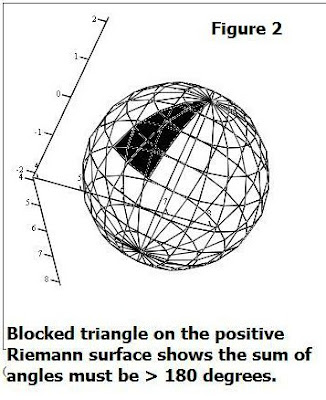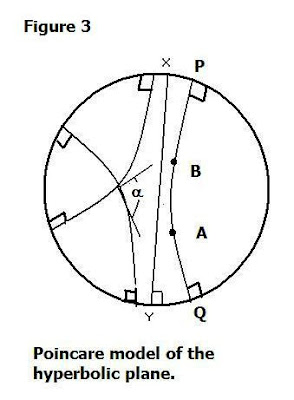Thursday, May 18, 2017

Math Revisited: Non-Euclidean GeometryMy first exposure to non-Euclidean Geometry occurred in late 1963, as I put the finishing touches on my science fair project's  model of a “dual” universe, consisting of matter and antimatter in dynamic equilibrium. I proposed the hypothesis that matter and anti-matter universes co-existed not only in a kind of energy equilibrium, but one of coupled space as well. Thus, each mass-energy component occupied a differently curved space-time, both being non-Euclidean. One of these (matter) had positive curvature and the space-time was Riemannian (after Bernhard Riemann), while the other (anti-matter) had negative curvature and the space time was Lobachevskian (after Janos Bolyai and Nicholai Lobachevsky – who developed it).

In my actual model I employed a transparent sphere for a postively curved - Riemannian geometry and inside it, enfolded within, a negatively –curved opaque space. Thus did I portray both antimatter and matter in one superspace or super-manifold. For convenience – the two spaces are depicted separately above (Fig. 1), the positive (Riemannian) on the left, and the negative (Lobachevskian) on the right.

As can be ascertained by inspection (looking carefully at the meridian circles and latitude parallels in the Riemannian sphere), there are no two parallel lines in the Euclidean sense, since any two geodesics (curves of shortest path) must intersect(see Fig. 2 below). Thus, the sum of the angles of the triangle formed by 3 geodesics will always total > 180 degrees. Looking at the spherical geometry, it’s also easy to see why positively curved Riemannian geometry was the first to be developed – because it was based on the already familiar geometry of the sphere. (From which we use spherical trigonometry to obtain distances and angles, e.g. using a law of sines of the form: sin a/ sin(A) = sin b/ sin (B) where common letters refer to sides and capital letters to angles.)

Conversely, the angles on a negatively curved surface formed by three geodesics, always total less than 180 degrees. Also, on a negatively curved surface both the circumference and area of a circle increase faster (with increasing diameter) than for Euclidean space (the converse holds for the positively curved space).

Of course, at the time I didn’t realize the proper terms for the respective spaces were: elliptic (for Riemannian or +-curved), and hyperbolic (for Lobachevskian or negatively curved space. Nor did I appreciate at the time  that all three geometries – Euclidean, elliptic and hyperbolic were actually related to each other via a model known as the Poincare disk (Fig. 3).In his disk model, Poincare depicted a hyperbolic plane within a Euclidean plane. The difference is that straight lines in Poincare’s disk once they are the same as geodesics (great circles) are warped. Thus, all straight lines on the disk appear to be curved lines – as shown – unless a particular line traverses the midpoint of the disk, in which case the apparent bending or warp is reduced to zero. So it appears as a straight line.

Another unique difference of the Poincare disk model from an ordinary circle is that the boundary of his model is infinitely far away or we would say “at infinity”. Another peculiar aspect concerns how right angles are formed and where. For example, the geodesic shown in the Poincare disk model makes right angles at either boundary. Similarly, any two geodesics that intersect (imagine another straight line 90 degrees different in orientation from that shown also passing through the disk center) form EUCLIDEAN angles at the point of their intersection.

Thus, the disk model is extremely valuable in that it permits discussion of non-Euclidean geometry in Euclidean terms. Let’s carry this forward looking at the curve PQ on the disk, and also the intermediary points A, B along it. Let’s assume we’d like to find the length x = AB, a segment of the curve PQ. Then one can show:

x = ln [(AQ/AP)/ (BQ/ BP)]

which uses a property common to natural logarithms called the ‘cross ratio’

Note the above definition contains embedded within the definition for points (A,B) , line (AB= x), length x, as well as “angle” – defined in terms of the Euclidean angle subtended at the point of intersection by tangents to the lines viewed as circular arcs in the putative Euclidean plane.

Let’s carry this forward looking at the curve PQ on the disk, and also the intermediary points A, B along it. Let’s assume we’d like to find the length x = AB, a segment of the curve PQ. Then one can show:

x = ln [(AQ/AP)/ (BQ/ BP)]

which uses a property common to natural logarithms called the ‘cross ratio’.  The above definition contains within it the definition for points (A,B) , line (AB= x), length x, as well as “angle” – defined in terms of the Euclidean angle subtended at the point of intersection by tangents to the lines viewed as circular arcs in the putative Euclidean plane.

. In the case of hyperbolic geometry the law of sines  takes a different one from that for spherical geometry (understandable given that the sum of angles in a hyperbolic triangle < 180 degrees!).  First, let’s recall the law of sines for the Euclidean case:
a/ b = sin(A)/ sin(B).

For the spherical case:

Sin A/ sin a = sin B/ sin b = sin C/ sin c

where A, B, C denote ANGLES and a,b,c denote measured arcs.

Now for the hyperbolic case we have:
sin (A)/ sin(B) = {(sinh(a/k)/ sinh(b/k)}

where A, B, a, b again have the standard definitions as per angles and sides, and k denotes a positive constant that is often confused with curvature.  To be more specific, the value of k depends on the choice of the units of measurement. The value of k also expresses a definite length, all other factors being equal.

For example, consider a number of concentric horocycles (such as QABP in Fig. 3) at a distance x from each other. Let their corresponding arcs have lengths: a1, a2, a3…..an. The ratio of each one to the next is then exp (x/k) where a1, a2, a3 etc. form a geometrical progression with the quotient:

exp (- x/ k) = 1  / (exp(x/k))

In other words, if the first arc is 1, the 2nd is 0.9, then the third is (0.9)2 = 0.81 and the fourth is (0.9)3  =  0.729 and so on.

For those who may not know, “sinh” defines the hyperbolic sine. From calculus texts this is defined based on the exponential function, exp(x) as:

sinh(x) = ½ (exp(x) – exp(-x))

with coordinates taken on the “unit hyperbola”: x2 – y2 = 1

Similarly, the hyperbolic cosine (cosh(x)) is defined:

cosh(x) = ½ (exp(x) + exp(-x))

In fact, when formulations are made one can obtain identities which parallel those of the standard trig functions. For example, in the latter we have:  cos2(x) + sin2(x) = 1, and in the hyperbolic sense one can prove:
cosh2(x) - sinh2(x) = 1

The hyperbolic cosine is useful to know when venturing into hyperbolic geometry because it is used to express a relation associating the 3 sides of a right triangle in hyperbolic space:
cosh(c/k) = cosh (a/k) cosh(b/k)

Many more detailed investigations of non-Euclidean geometry are possible, and also seeing how they link up with Euclidean geometry in a more general formalism. The basis for doing this entails taking a more comprehensive analytic (e.g. 'manifold') approach,  wherein we associate lines and points with a geometry. A simplified form for this may be expressed using the matrix formulation in 4(a).Where the left side denotes a line [u1, u2, u3]. If one finds that the point specified by (x1, x2, x3)  is the same as for another point specified (y1,y2, y3) such that the matrix relation in 4(b) is true then we can say that the correlation constitutes a polarity. It is possible that a suitable choice of polarity can be found for any combination of points for a line [u1, u2, u3] such that: u1 = x1, u2 = x2 and u3 = cx3.  In this case, if c = +1, the geometry is elliptic (e.g. Riemannian), and if c =-1 it is hyperbolic.   This gets us into the area of projective geometry.

Practice  Problems:

1. Consider a triangle in hyperbolic space as computed by an interstellar ship traversing it. The dimensions are: a = 1 pc, b = 2 pc and c is unknown where pc denotes parsec (1 pc = 3.26 light years).  Using this and the equation associating the 3 sides of a right triangle in hyperbolic space, with k = 1, find the dimension of c. Thence, find the ratios of the sines of the angles, e.g. (sin A/ sin B)

2. Consider the Lobachevskian surface shown in Fig. 1.  Assume  it displays concentric horocycles such that exp (- x/ k) =      1 / (exp(x/k)) and when the ratios for x/k are taken the progression is of the form: 1, 0.5, 0.25, 0.125 etc.  Use this to deduce the curvature k, if k = -1/ k2.  Is the non-Euclidean surface shown above a Riemannian or Lobachevskian space? Give reasons.